Next: Output negative digits Up: Division Previous: Output zero case:

#### Output positive digits

Positive digits are output if the numerator x is of the form (1::0::x') or (1::1::x'). If so, we can immediately say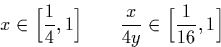The obvious strategy here would be to generate the digit one (1) in the output stream. Unfortunately if we do so it apparently becomes very complicated to express the remainder of the result as a recursive call (doing so would probably require examination of more digits of the input streams x and y). An algorithm which outputs signed binary digits must exist as the representations used are all equivalent, but would probably be significantly more complex than the one presented here and require a larger lookahead.

The solution is to output a dyadic digit instead of a signed binary one, and to determine which digit by considering the result r of subtracting the denominator y from the numerator x. Let

r = y-x

Now, we examine the first two digits of r. There are three different possible cases to consider:

Case 1:
The result r starts (1::1) or (1::0). Hence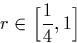We can deduce further that as x can be no greater than 1, and y is no less than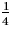, r can be no greater than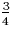. Similarly,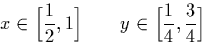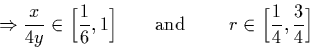Observe now that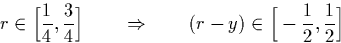So we can represent the value r' = 2(r-y) = 2(x-2y) in a stream. Now:

Case 2:
The result r starts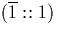, , or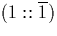. Therefore: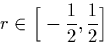And we can represent r' = 2r = 2(x-y) in a stream. Now:

Case 3:
The result r starts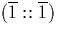or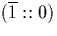. Hence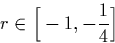We can deduce further that as x can be no less than, and y is no greater than 1, and therefore r can be no less than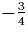. Furthermore we can deduce: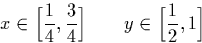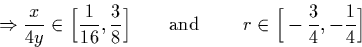We cannot use r to express the result in terms of a digit and a recursive call directly, so we define r' as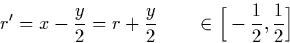As 2r' is in [-1,1], we can represent r'' = 2r' = 2x - y in a stream. Now, we can find a recursive call as follows:Next: Output negative digits Up: Division Previous: Output zero case:
Martin Escardo
5/11/2000